## Multiplying and Analog Computation

Analog arithmetic is easy to do, and we also double frequency and measure noise power

### The Multiplier

We have already encountered multiplication of two signals, in Amplitude Modulation and Superheterodynes, where an AM signal is produced by multiplying the modulation and the carrier, to produce the sidebands, and adding enough additional carrier so that the envelope reproduces the modulation, allowing the modulation to be recovered easily by rectification and filtering. In this case we used a balanced modulator, which is the fundamental multiplying element.

Another application is finding power, which is the product of the voltage and the current. If we produce a voltage proportional to the current, then the power is the product of two voltages. It is possible to adapt a balanced modulator to this purpose, but it is quite involved to handle the various voltage levels and to find an output that is proportional to the product of the inputs over a practical range. There is an easier way: the analog multiplier integrated circuit, where all this has been handled for us. The ouput of this circuit should be the product of its input voltages, with convenient ways to handle different voltage references.

The Burr-Brown type MPY634KP, in a 14-pin DIP, does all this very nicely. This is a relatively expensive chip, but is worth it for all the bother it saves, and for the wonder of owning such a fascinating device. This is an industry-standard device, also available from Analog Devices, which offers the less-expensive AD633 as well. The MPY643 is a high end device with great flexibility. It multiplies from DC to 10 MHz, so it is capable of handling most multiplication you may have in mind. As soon as you apply power, ±12 V, it is ready to multiply with a simple hookup and no external parts. The output of the circuit is from a high-gain (85 dB) op-amp, so it is necessary to close the feedback loop, which is done with a connection from the output to the Z1 input. A voltage divider will permit you to change the gain. Now the chip will give you (X1 - X2)(Y1 - Y2)/10 + Z2 at the output, pretty nearly. The 10 is the scale factor SF, so that the output does not exceed 10 V for inputs in the range of ±10 V (with Z2 = 0). The SF pin is used to adjust the value of SF from 3 to 10 V, by connecting a resistor to the negative supply. Since the chip operated well leaving this pin disconnected, I did so.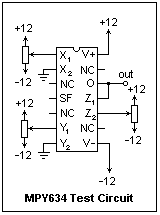A circuit for exercising the MYP634 is shown at the right. Input voltages must never leave the range +V to -V. This is guaranteed by the way the pots are connected. 10k pots are satisfactory, but the value is not important. The inputs X, Y and Z are high impedance. The output can be short-cicuited to ground, but will supply about 30 mA when necessary. As you can see, the connections are very straightforward. It is a convenience to have a lot of DMM's for the tests! Verify that the chip gives the correct sign for any signs of the inputs X and Y. This means that it is a four-quadrant multiplier, the most general type. A two-quadrant multiplier takes one input as positive, while a one-quadrant multiplier has both inputs and the output positive.

It is really pleasant to see the chip multiplying with time-dependent signals. Take a 10k resistor with one end grounded, and put an alternating voltage of 1 kHz or so across it with a function generator through a coupling capacitor (perhaps 1 μF plastic). Use the scope to be absolutely sure the voltage does not exceed ±12 V when it is connected to the multiplier. Now connect X and Y to the top of the resistor, and Z2 to ground. The output of the multiplier should then be V2/10. This is precisely the instantaneous power in the resistor in mW, since the 10 accounts for the 10k in converting current to voltage. Display the input voltage and the output of the multiplier on the scope, with the DC connection. The power will vary sinusoidally at twice the frequency, always positive. Check its value to see that the display is really in mW. Note the phase relations as well. The maximum powers for positive and negative loops are not quite equal (capacitive coupling eliminates any DC component), which means the chip has a slightly greater gain in the first quadrant than in the third quadrant (difference of 5%). This seems to violate the specifications (0.4% nonlinearity), but is not very bad. I suppose they do mainly government work at Burr-Brown.

Now put an 0.01 μF capacitor in series with the 10k resistor, and connect X to the top of the capacitor, leaving Y connected to the resistor. You can keep the coupling capacitor or not; if not, adjust the input to have a zero DC component. Now you are measuring the product of the voltage across an RC circuit with the current through it. The average power should be VI cos θ, while the instantaneous power should vary at twice the voltage frequency. Find what everything should be by analyzing the circuit, and check that this is displayed on the oscilloscope. For part of the cycle, the power is actually negative, when the capacitor is supplying power in the reverse direction. This exercise is quite valuable and entertaining for perfecting one's understanding of AC circuits, and has many variations--for example, with resonant circuits.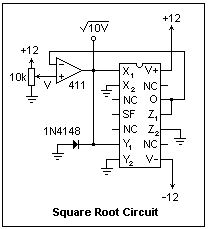If you can produce a function, you can also obtain its inverse. The method is shown in the circuit on the right, which takes the square root of an input voltage. The output of the multiplier is fed back to the inverting input of an op-amp, which then strives to make this voltage equal to the voltage applied to its noninverting input. When it gets the correct input, you can pick it off at the output of the op-amp for your purposes. A similar circuit will perform division. The diode is there to "catch" the op-amp if you try to give it an impossible job, like the square root of a negative number. Then it goes to negative saturation if not prevented. This circuit worked fairly well, but did not give exact results. Perhaps a little trimming of SF would cure this problem. Note how the factor of SF = 10 enters; the output of the op-amp is x2/10, which is compared with the input.

The multiplier can be the basis of a wattmeter giving the actual average power for any waveform, or "true RMS," not simply an estimate based on sinusoids or other approximations. If you use the MPY634 for amplitude modulation, the modulation percentage is controlled either by the DC level of the modulation input, or by extra carrier fed to Z2 via a potentiometer. A multiplier can also serve as a Type I phase detector in a phase-locked loop. If one of the inputs is a constant voltage, it sets the gain for the other input, so a voltage-controlled amplifier results.

### A Frequency Doubler and a Divider

As an exercise with the Analog Devices AD633 multiplier, let's make a frequency doubler. There are various ways of doing this, but the most ingenious, taken from Analog Devices data sheets, is to use the trigonometric relation 2 sin θ cos θ = sin 2θ. We need to get the sine and cosine of the fundamental frequency, and then multiply them. The sine and cosine have the same shape, but are 90° apart in phase. If we take the input signal and shift it by +45° and -45° using an RC circuit, we will have the desired sine and cosine.The circuit is shown at the right. Note that only one RC circuit is necessary, since we have both inverting and noninverting inputs available for each factor. R and C must be chosen to suit the operating frequency, but the doubler is fairly broadband, and will work over a range of frequencies. For 1kHz, use R = 16k and C = 0.01μF. The 1k and 3k resistors on the ouput are for adjusting the amplitude. Using 4.7k instead of 3k, I got input and output amplitudes equal at 15 V peak-to-peak. The DC bias in the input must be carefully adjusted to zero by looking at the output waveform, which is symmetrical when the bias is zero. Pin 7 is the output of the output amplifier, while pin 6 is the Z input, which is added to the output. The function of the AD633 is expressed as V = (X1 - X2)(Y1 - Y2)/10 + Z. Pin 1 is X1, pin 2 X2, pin 3 Y1 and pin 4, Y2. The power supply can be ±8 to ±18 V, nominally ±15 V. The power connections can be bypassed with 0.1 μF capacitors, which are not shown in the diagram. This circuit will only double the frequency of a sine wave, of course, and will do strange things with other waveforms.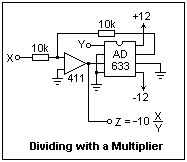A circuit for dividing with the AD633 is shown at the left. Analog Devices recommends an AD711 op-amp, but the 411 should do as well. The MPY634 could be used as well, as mentioned above in connection with square roots. In general, if you can perform the direct function, the inverse function can also be obtained by using an op-amp. Note that there is the possibility of scaling the X input by choosing the feedback resistors in this circuit.

The AD633 has fewer connections than the MYP634, so it is even easier to use--simply connect the power and it's ready to go. It is slightly less adaptable, but this is no disadvantage in most applications. It is also cheaper, which is a consideration. The analog multiplier is a versatile device that should be kept in mind.

### RMS Conversion

The average level of a varying signal can be expressed in terms of its peak value Vp, the full-wave rectified average value Vav, or the rms value Vrms, which is the square root of the average value of the square of the signal. For a signal of determinate waveform, all these values are proportional. The ratio Vp/Vrms is called the crest factor, and the ration Vrms/Vav is called the form factor. For a sine wave, these ratios are 1.414 and 1.11, respectively, for a triangle wave 1.732 and 1.15, and for a symmetrical square wave, both are unity. It is easy to verify these figures for yourself, an exercise that will give a better comprehension of what they mean.

If the waveform is not periodic, but still can be described statistically, these factors may be constant on the average, so the various measures can be related to one another. With Gaussian noise, for example, the crest factor is 3.0, a good rule of thumb for determining the energy content of a noise signal observed on an oscilloscope. In the general case, however, none of the measures are reliably related to one another and depend on the actual waveform. In this case, only the rms value is a good statistic, with its square proportional to the average power.

The usual way a DMM determines the rms value of an AC signal is to full-wave rectify and filter the signal, then calibrate the readout so that this average value is multiplied by 1.11. This works fine on sinusoidal signals, and also on signals that are not too far from sinusoidal. Even for a triangle wave, the result is only 4% too low, but for a square wave it is 11% too high. Good enough for government work, obviously, but not for careful work with strange waveforms or noise. Here we need to find the "true" rms value, rather than one calculated from the average value.

Analog Devices supplies a chip that will do just this, the AD736. There are more costly premium chips as well, but the AD736 is completely adequate for the job. It uses a bipolar power supply ranging from a low of +2.8,-3.2 V to ±16.5 V. It works well on ±5 V, which I used. If you do not have a suitable supply, then use ±12 V. Bypass both supplies with 0.1μF capacitors to ground to prevent noise from entering here. There are two inputs to the input op-amp of the device, pin 1 (low impedance, inverting) and pin 2 (high impedance, noninverting). If you use pin 1, send the signal through a coupling capacitor, perhaps 10μF, and ground pin 2. If you use pin 2, pin 1 can be left open, or, better, grounded through a 10μF capacitor. Protect the high-impedance input with a pair of diode clamps and a 47k resistor, as shown in the circuit. This prevents signals outside of the power supply range from damaging the chip. Any signal diode can be used, though the common 1N4148 is shown.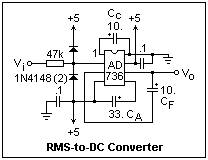A complete circuit is shown at the right. Inside, the chip contains a full-wave rectifier. If you do not connect the capacitor CA, the output will be averaged by filter capacitor CF to give Vav, which you can multiply by any constant you wish using an op-amp. There is also an RMS conversion unit, and if you supply an averaging capacitor CA, the output will be proportional to Vrms. Then, CF, which is optional, serves to smooth the output. The values of the capacitors determine the averaging times and bandwidths, as is obvious. The values shown here are good for general service. CA can range up to 150μF, and CF up to 100μF, if desired. The AD736 will handle crest factors up to 5.

I made measurements on the output of my function generator, which supplied square, triangle and sine waves. I adjusted the average (DC) value of the output to 0, although the AD736 did not seem to be sensitive to the DC level and did not include it in the rms value (I do not fully understand why not). I presumed that the peak values of each of the three waveforms was the same, a rather coarse assumption, and calibrated the output with the square wave. Adjusting the average value to 0 with a DMM, I found outputs of 2.435 V, 1.476 V and 1.691 V for square, triangle and sine waves, respectively, using a DMM on the DCV function. This gave crest factors of 1.440 (ideally 1.414) and 1.650 (ideally 1.732), which shows that the converter is working. It seems to work better for values less than 1.0 V. The frequency was 1 kHz. If you apply a 2 V peak-to-peak sine wave, the output should be about 0.707 V. It would be interesting to observe the effect of varying the frequency. If the low-impedance input is used, the data sheets promise a rather wide bandwidth, over 100 kHz.

### Noise Power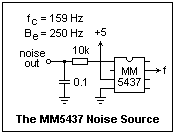The AD736 is excellent for measuring the rms voltage of a noise signal. A source of band-limited white noise is shown at the right, which uses an MM5437 noise source chip. This is a 23-stage shift register with feedback from the 18th stage, producing a pseudorandom sequence of 1's and 0's that, when filtered, gives analog band-limited noise. The simple RC filter shown has a noise-equivalent bandwidth of 1/4RC = 250 Hz. At low frequencies, the MM5437 produces a noise power of about 2.0 x 10-5 V2/Hz, so the noise power output of this source will be about 5.0 x 10-3 V2. The square root of the noise power is the rms noise voltage, which should be about 71 mV here. Note that "noise power" is not really power, but voltage squared, since it is voltage squared that adds at different frequencies.

All you have to do to get the MM5437 to work is to apply the power, from 4.5 V to 11 V. I have used 5 V here, which is consistent with the usual logic supply voltage. At pin 7 a square wave appears, which is called the "clock frequency." For my chip, the clock frequency was 174 kHz. The amplitude of the unfiltered output can be measured on the oscilloscope to be about 3.7 V. The usual formula for the power spectral density of this random telegraph waveform is 2a2/f, where a is the half-amplitude, or 1.85 V here. To agree with the power spectral density that we will measure, one must use twice the measured clock frequency. Apparently, the frequency output is the output of a flip-flop driven by the shift clock, and so is at half frequency. With this agreement, we get the power spectral density quoted above.

Apply the filtered noise to the AD736. I found the output voltage to be about 70 mV. Note that this voltage fluctuates, as expected for a short averaging period. Next, I used a "true-rms" DMM to measure the noise output of the MM5437, and read 60 mV. Finally, I observed the noise signal on the oscilloscope, and found that it fit within a 400 mV band. Since the peak factor for Gaussian noise is 3, the rms voltage can be estimated at 400/6 = 66 mV. All these figures agree, if not exactly. The form factor for Gaussian noise seems to be about 1.24, so we could also rectify it and apply this factor for a fourth estimate.

If pin 8 is tied low, an external clock can be applied to pin 7 for clocking the shift register at any desired rate. Also, if pin 6 is tied low, the shift register can be loaded with data applied at pin 5 and clocked in. These features are needed only in quite special applications. Pin 2, by the way, is a no-connection.

### Analog Computers

It has long been appreciated that electrical circuits have close analogies with mechanical and other systems, where the voltages or currents represent analogous variables in the systems represented. By measuring voltages or currents, one can simulate the behavior of systems that might be too complicated for accurate numerical calculation. In fact, such analog computers were once actually practical, although the more recent vast expansion in digital computation has rendered them obsolete for solving general problems. In spite of this, analog computation still has applications (as in the true power measurement mentioned above), and besides is intrinsically interesting.

The typical analog computer used voltages between +10 and -10 V to represent its variables. The greatest drawback of analog computation appears is the severe lack of dynamic range. Digital computers can use floating point to escape this limitation, but there is nothing similar for analog computation, so the problems must be carefully devised. We should be able to add, subtract and multiply by constants these voltages, and it is easy to see how to carry out these operations with op-amps. In fact, op-amps got their name in this way, and analog computation was their first application. Such devices are called adders.

Differential equations involve derivatives, but analog differentiation is an unstable operation. Differentiation is replaced by integration, which is stable and easily accomplished by an op-amp with a capacitor in its negative feedback loop. These operational integrators must be capable of being set to precise initial values that represent the initial conditions for the differential equations involved. This means that the analog computer gives a particular solution of a differential equation. An analog computer can easily solve systems of simultaneous linear differential equations with constant coefficients using only integrators and adders. Such equations can, of course, be solved by digital computation, which is the method of choice now. It is, however, very pleasant to see the solution actually developing in time.

To set up a problem appropriately for integrators, it is convenient to represent differentiation by -jω, then to divide by this factor until it appears only in denominators. Then it can be replaced by integration. Initial values are considered when fixing the limits of integration, which are usually 0 and t. Multiplication by constants may be necessary to keep variables within bounds in simultaneous equations. Once a problem is set up, different initial conditions, and different forcing functions may be studied easily. Constants, represented by potentiometers, can be adjusted to see what effects result.

The biquad filter is a small analog computer with two integrators and an adder that solves a simple second-order differential equation representing a filter or other interesting kind of response. It can be connected in several ways to give different kinds of systems, such as lowpass, highpass, bandpass and notch. Digital computation is fast enough to actually be used this way, with digital signal processors, but the biquad filter is a simpler and cheaper solution in many cases. We had an example in Switched Capacitors and Filters, the MF10 package.

The multiplier is another operation that opens the wide field of nonlinear systems. Such systems may not be so easily solved digitally, and straightforward analog computation may be quite convenient. The MPY634 is ready to go for this application, and needs little support or additions. By adding op-amps, the multiplier can also divide and take square roots.

The physical analog computer consists of the power supplies, and places to plug in the operational units, which are then connected appropriately by patch cords. There are facilities for providing fixed voltages for initial conditions, and circuits to initialize and release the integrators at t = 0. The variables can be observed on oscilloscopes or meters, or the values can be captured by analog to digital conversion. In fact, a small digital computer would do very well in managing an analog computer.

### References

Burr-Brown Application Bulletin AB-083 deals with the MPY634, and is available from Burr-Brown. Since Burr-Brown has merged with TI, there is some confusion with the website, but use the search facility to locate the AB, which can be downloaded as an Acrobat file.

The Analog Devices AD633 and AD736 can be obtained from Newark (see Your Laboratory for a link) for about \$11 each. Data sheets are available in PDF format at Analog Devices.